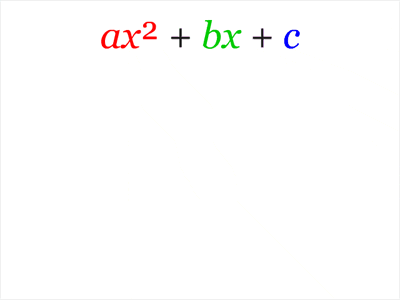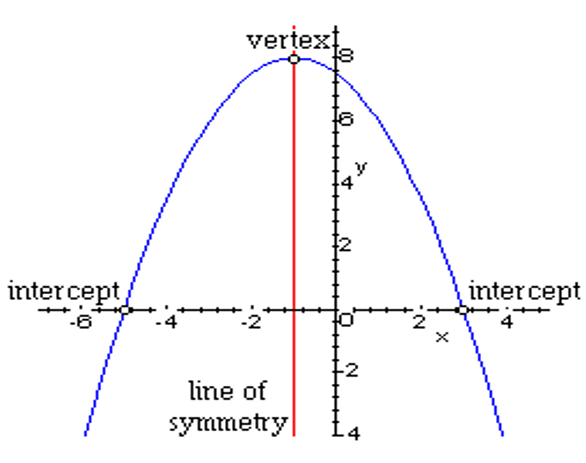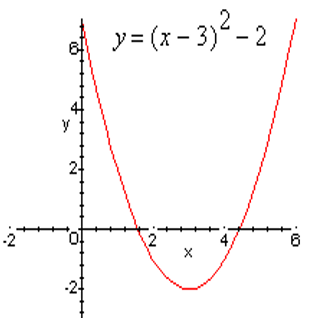Mathematics

# How do you solve quadratic equations?

77 views

 1 Introduction 2 General Form 3 How to find the zeros of a quadratic function 4 Summary 5 FAQs

20 October 2020

## Introduction

The word “quadratic” came from “quadrature”, the Latin word for square. Hence, we can define a quadratic equation as an equation where the variable is of the second degree. Therefore, a quadratic equation is also called an “Equation of degree 2”.

Consider this example. Suppose you want a plot of land and want to build a house there.

Assume the area of the plot is 300 m2. You want to make your house have a breadth equal to twice its length. Let $$‘x’$$ be the length then breadth $$= 2x.$$ Also, we might have Length×Breadth = Area of the House.How will you optimize the area of land you have? We can write the area of the house as $$(x) × (2x) = 2x^2.$$ If $$2x^2 = 300,$$ we will have optimized the area available to us.

This is called a quadratic equation. Solving for $$x$$ gives us the value of $$x = 12.247 \text{ m}.$$So to make optimal use of the land, we just have to make the length of our house $$= 12.247 \text{ m}$$ and the breadth $$= 24.495 \text{ m.}$$

## General form of Quadratic Equation

### The Standard Form

We must know that a quadratic polynomial can be written as $$ax^2 + bx + c.$$ This is known as the general form or quadratic form.

If the quadratic polynomial = 0, it forms a quadratic equation. Therefore, the standard quadratic form of a quadratic equation can be written as: $$ax^2 + bx + c = 0;$$ where x is an unknown variable, and a, b, c are constants with ‘a’ ≠ 0 (if a = 0, then it becomes a linear equation).Source: giphy.com

The constants ‘a’, ‘b’, and ‘c’ are called coefficients. Let us look at some examples of a quadratic form:

• $$2x^2+5x+3=0;$$ In this, a=2, b=3 and c=5
• $$x^2-3x=0;$$ Here, a=1 since it is 1 times $$x^2,$$ $$b=-3$$ and $$c=0,$$ not shown as it is zero.

But sometimes, the quadratic equation does not come in the standard quadratic form. These are the hidden quadratic equations that we might have to reduce to the quadratic form. Here are some examples:

 Equation Standard Form Coefficients Explanation x2 – 3x = 1 x2 – 3x – 1 = 0 a = 1, b = -3, c = -1 Compare it to the general form of the quadratic equation and subtract 1 from both sides. 2(z2 – 2z)=5 2z2 – 4z – 5 = 0 a = 2, b = -4, c = -5 We need to expand (open the brackets)  by multiplying 2 with z2 and -2z and also we need to bring 5 to the left side to equate the equation with 0. y(y-2)=0 y2 – 2y = 0 a = 1, b = -2, c = 0 We need to expand, multiply y with both y and -2 and the output you get is in the desired general form.

### How to write a quadratic function in standard form?

The functions above are examples of quadratic functions in standard quadratic form. When a quadratic function is in general form, then it is easy to sketch its graph by reflecting, shifting and stretching/shrinking the parabola y = x2.

The quadratic function $$f(x) = a(x - h)^2 + k,$$ not equal to zero, is said to be in standard quadratic form. If a is positive, the graph opens upward, and if a is negative, then it opens downward.

The line of symmetry is that the vertical line $$x = h,$$ and therefore the vertex is that the point (h,k).

So, how to write a quadratic function in standard form?

Any quadratic function is often rewritten in standard quadratic form or general form by completing the square. Note that when a quadratic function is in its general form it's also easy to seek out its zeros by the root principle.

Let us now look at some examples to fully understand how to write a quadratic function in standard form.

 Example

Consider this in its general form.

$$f(x) = -2x^2 + 2x + 3$$

Find the vertex of the graph of f.

Solution

\begin{align}f(x) &= -2x^2 + 2x + 3\\&= (-2x^2 + 2x) + 3\\&= -2(x^2- x) + 3\\&= -2(x^2 - x + \frac{1}{4} -\frac {1}{4}) + 3\end{align}

We add and subtract $$\frac{1}{4},$$ because $$(\frac{-1}{2})2 = \frac{1}{4},$$ and -1 is that the coefficient of x.

$$= -2(x^2 - x + \frac{1}{4}) -2(-\frac{1}{4}) + 3.$$

Note that everything within the parentheses is multiplied by -2, so once we remove $$-\frac{1}{4 }$$from the parentheses, we must multiply it by -2.

\begin{align}f(x) &= -2(x -\frac{1} {2})2 + \frac{1}{2} + 3\5pt]&= -2(x - \frac{1}{2})2 + \frac{7}{2}\end{align} . The vertex is the point $$(\frac{1}{2}, \frac{7}{2})$$. So the graph opens downward $$(-2 < 0),$$ the vertex is the highest point on the graph. ## How to find the zeros of a quadratic function? In pre-calculus, you might have used the zero-product property to find the roots of a factored equation. After you factor a polynomial into its different sets, you can identify each set equal to zero to solve for the roots with the zero-product property. The zero-product property predicts that if several factors are multiplying to give you zero, at least one of them has to be zero. Your work is to find all the values of x that make the polynomial equal to zero. If the polynomial is factored as you can set each factor equal to zero and solve for x. So, how to find the zeros of a quadratic function? Factoring $$x ^2+ 3x – 10 = 0$$ gives you $$(x + 5)(x – 2)$$. Moving ahead is easy because each factor is linear (first degree). The equation $$x + 5 = 0$$ gives you a solution, $$x = –5,$$ and $$x – 2 = 0$$ gives you the other solution, $$x = 2.$$ These answers each become an x-intercept on the graph of the polynomial. Sometimes after you’ve factored, one or both of the two factors can be factored again, in this case, you should continue factoring. In other cases, they may be non factorable. If one of these factors is quadratic, you could find the roots only by using the quadratic form. For example, $$6x^4 – 12x^3 + 4x^2= 0$$ factors to $$2x^2(3x^2– 6x + 2) = 0.$$ The first term, $$2x^2 = 0,$$ is solvable using algebra, but the second factor, $$3x^2– 6x + 2 = 0,$$ is non factorable and requires the quadratic form. In other cases, they may be non factorable, in which case you can solve them only by using the quadratic form.  Example Consider $$f(x) = 3x^2 + 12x + 8$$ in general form. Sketch the graph of f ,find its vertex, and find the zeros of f. AnswerAn alternate method of finding the vertex In some cases completing the square isn't the simplest thanks to finding the vertex of a parabola. If the graph of a quadratic function has two x-intercepts, then the road of symmetry is the vertical line through the midpoint of the x-intercepts. The x-intercepts of the graph above are at -5 and three. The line of symmetry goes through -1, which is the average of -5 and three. $$\frac{(-5 + 3)}{2} = \frac{-2}{2} = -1$$ Once we all know that the road of symmetry is $$x = -1,$$ then we all know the primary coordinate of the vertex is $$-1.$$ The second coordinate of the vertex is often found by evaluating the function at $$x = -1$$  Example Consider the function $$f(x) = x^2 - 6x + 7$$ in general form. Sketch the graph of f and find its zeros and vertex. Solution $$f(x) = x^2 - 6x + 7$$ $$= (x2 - 6x )+ 7.$$ Group the $$x^2$$ and x terms then complete the square on these terms. $$= (x^2 - 6x + 9 - 9) + 7$$ We need to feature 9 because it's the square of 1 half the coefficient of x, $$(\frac{-6}{2})2 = 9.$$ Hence we were solving an equation. We simply added 9 to each side of the equation. In this setting, we add and subtract 9 in order that we don't change the function. $$= (x^2 - 6x + 9) - 9 + 7$$ We see that $$x^2 - 6x + 9$$ is a perfect square, namely $$(x - 3)^2.$$ $$f(x) = (x - 3)^2 - 2$$ This is the standard form. From this result, one easily finds the vertex of the graph of $$f$$ is $$(3, -2).$$ To find the zeros of $$f,$$ we set f adequate to 0 and solve for $$x.$$ \[\begin{align}(x - 3)^2 - 2 &= 0\\[5pt](x - 3)^2 &= 2\\[5pt](x - 3) &= ± \sqrt{2}\\[5pt]x &= 3 ± \sqrt{2}\end{align}

To sketch the graph of f we shift the graph of $$y = x^2$$ three units to the proper and two units down.If the coefficient of $$x^2$$ isn't 1, then we must factor this coefficient from the $$x^2$$ and $$x$$ terms before proceeding.

## Summary

In this section, we learned how to factor a quadratic form, how to find the zeros of a quadratic function and how to write a quadratic function in standard form. Here, we factor quadratic equations of the quadratic form $$x^2 + bx + c = 0,$$ splitting the expression into two binomials and using the zero product property to solve the quadratic equation.

Not all equations $$ax^2 + bx + c = 0$$ can be easily factored. Thus, we need a general form to solve for $$x.$$ This is the standard quadratic form and we use it as a reference.

Written by Gargi Shrivastava

## What are the roots of the quadratic function $$f(q) = q^2 – 125?$$ check all that apply $$q=+5\sqrt{5}$$ and $$q=-5\sqrt{5}$$

Solving:

$$f(q)=q^2-125$$

To determine the roots of the given quadratic equation

we can replace $$f(q)$$ with 0 and solve for q

$$0=q^2-125$$

We can add 125 on both sides

$$125=q^2$$

We can take square root on both sides $$q=+-5\sqrt{5}$$

$$q=+5\sqrt{5}$$ and $$q=-5\sqrt{5}$$

The given options are not roots of q(x)

## What are the zeros of the quadratic function $$f(x) = 6x^2 + 12x – 7$$?

$$x = \frac{1}{4} = 0.25$$ and $$x =\frac{-9}{4} = -2.25$$

Solve:

$$−6x+12x−7=0$$ is a quadratic equation in standard form: $$ax^2 +bx+c$$, where $$a=−6, b=12,$$ and $$c=−7.$$

The quadratic form can be used to solve this equation.

$$x=−b±\sqrt{b2}−4ac2a$$

Substitute the given values into the formula.

\begin{align}x&=−12±\sqrt{122}−4×−6×−72×−6\\x&=−12±\sqrt{144}−168−12\\x&=−12±\sqrt{−24}−12 \end{align}

Factor −24.

\begin{align}x&=−12±\sqrt{2}×2×2×3i−12\\x&=−12±2\sqrt{6i}−12\end{align}

Simplify by dividing by −12.

$x=1±2\sqrt{6i}6$

Solutions for x.

$$x=1+2\sqrt{6i6},\,1−2\sqrt{6i6}$$

## Which is a zero of the quadratic function$$f(x) = 16x + 32x − 9$$?

Solve:

$$f(x) = 16x^2 + 32x - 9$$

To find the "zeros", set $$f(x) = 0$$:

$$0 = 16x^2 + 32x - 9$$

The equation is solvable by factoring or by using the quadratic form. The factors (use the "ac" method) are:

$$0 = (4x-1)(4x+9)$$

$$x = 1/4 = 0.25$$ and $$x = -9/4 = -2.25$$

## What are the zeros of the quadratic function $$f(x) = 2x^2 + 16x – 9$$?

$$x =\frac{(16+328)}{-4}=4-\frac{1}{2}\sqrt{ 82}= -8.528$$

or:

$$x =\frac{(16-\sqrt{328})}{-4}=4+\frac{1}{2}\sqrt{ 82} = 0.528$$

Solving

$$-2x^2-16x+9 = 0$$ by the Quadratic Form .

According to the Quadratic Form, x , the solution for $$Ax^2+Bx+C = 0$$ , where A, B and C are numbers, often called coefficients, is given by :

\begin{align}x=\frac{- B ± \sqrt{B2}-4AC}{2A}\end{align}

In our case,
$$A = -2$$

$$B = -16$$

$$C = 9$$

Accordingly,

$$B^2 - 4AC =$$

$$256 - (-72) =328$$

\begin{align}x=\frac{16 ± \sqrt{328}}{-4}\end{align}

Can $$\sqrt{328}$$ be simplified?

Yes! The prime factorization of 328 is

$$2•2•2•41$$

To be able to remove something from under the radical, there have to be 2 instances of it (because we are taking a square i.e. second root).

$$\sqrt{328} = \sqrt{2•2•2•41}=± 2 • \sqrt{82}$$

$$\sqrt{82},$$ rounded to 4 decimal digits, is 9.0554

So now we are looking at:

$$x = \frac{(16 ± 2 9.055 )}{-4}$$

Two real solutions:

$$x =\frac{(16+\sqrt{328})}{-4}=4-\frac{1}{2}\sqrt{82} = -8.528$$

or:

$$x =\frac{(16-\sqrt{328})}{-4}=4+\frac{1}{2}\sqrt{82} = 0.528$$

## Which is a zero of the quadratic function $$f(x) = 16x^2 + 32x − 9$$?

$$x= -2.5$$ and $$x= 0.5$$

Related Articles
GIVE YOUR CHILD THE CUEMATH EDGE
Access Personalised Math learning through interactive worksheets, gamified concepts and grade-wise courses